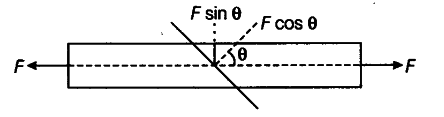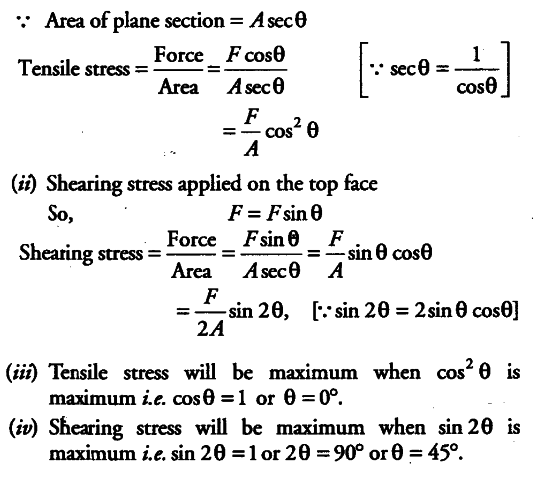# A bar of cross-section A is subjected to equal and opposite tensile forces at its ends

A bar of cross-section A is subjected to equal and opposite tensile forces at its ends. Consider a plane section of the bar whose normal makes an angle 0 with the axis of the bar.
(i) What is the tensile stress on this plane?
(ii) What is the shearing stress on this plane?
(iii) For what value o/0 is the tensile stress maximum?
(iv) For what value o/0 is the shearing stress maximum?The resolved part of F along the normal is the tensile force on this plane and the resolved part parallel to the plane is the shearing force on the plane.